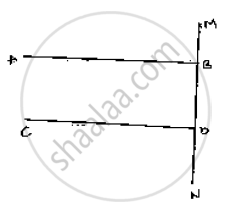# If Each of the Two Lines is Perpendicular to the Same Line, What Kind of Lines Are They to Each Other? - Mathematics

If each of the two lines is perpendicular to the same line, what kind of lines are they to each
other?

#### SolutionLet AB and CD be perpendicular to MN

∠ABD = 90°       [ AB ⊥ MN ]             ....(i )

∠CON = 90°      [CD ^ MN ]             ....(ii)

Now,

∠ABD = ∠CDN = 90°                 [From (i) and (ii)]

AB parallel to CD,

Since corresponding angle are equal

Is there an error in this question or solution?
Chapter 10: Lines and Angles - Exercise 10.4 [Page 47]

#### APPEARS IN

RD Sharma Mathematics for Class 9
Chapter 10 Lines and Angles
Exercise 10.4 | Q 8 | Page 47

Share